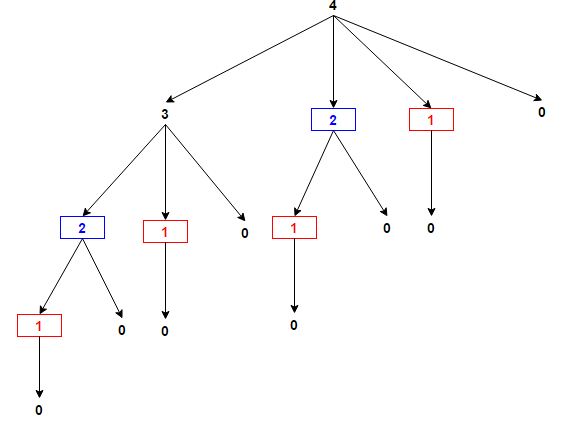# Maximum Product Rod Cutting

Given a rod of length n, find the optimal way to cut rod into smaller rods in order to maximize product of price of each of the smaller rod. Assume each rod of length i has price i.

For example, consider below rod of length 4

Best: Cut the rod into two pieces of length 2 each
to gain revenue of 2*2 = 4

Cut               Profit
4                  4
1, 3              (1 * 3) = 3
2, 2              (2 * 2) = 4
3, 1              (3 * 1) = 3
1, 1, 2           (1 * 1 * 2) = 2
1, 2, 1           (1 * 2 * 1) = 2
2, 1, 1           (2 * 1 * 1) = 2
1, 1, 1, 1        (1 * 1 * 1 * 1) = 1

Similarly for n = 6, (3 * 3) = 9
For n = 8, (2 * 3 * 3) = 18
For n = 15, (3 * 3 * 3 * 3 * 3) = 243

The idea is very simple. We partition the given rod of length n into two parts of length i and n-i for each 1 <= i <= n. Then we recurse for rod of length n-i but don’t divide rod of length i any further. Finally, we take maximum of all values. This yields the below recursive relation –

rodcut(n) = max { n, i * rodCut(n – i) } where 1 <= i <= n

Below is C++ and Java implementation of the idea:

## C++

Output:

Maximum Profit is 243

## Java

Output:

Maximum Profit is 243

The time complexity of above solution is O(nn) and auxiliary space used by the program is O(1).

We have seen that the problem can be broken down into smaller subproblems which can further be broken down into yet smaller subproblems, and so on. So the problem has an optimal substructure. Let us consider recursion tree for rod of length 4.As we can see, the same sub-problems (highlighted in same color) are getting computed again and again. So the problem also exhibits overlapping subproblems. We know that problems having optimal substructure and overlapping subproblems can be solved by dynamic programming, in which subproblem solutions are Memoized rather than computed again and again.

We will solve this problem in bottom-up manner. In the bottom-up approach, we solve smaller sub-problems first, then solve larger sub-problems from them. The following bottom-up approach computes T[i], which stores maximum profit achieved from rod of length i for each 1 <= i <= n. It uses value of smaller values i already computed.

Below is C++ and Java implementation of the idea:

## C++

Output:

Maximum Profit is 243

## Java

Output:

Maximum Profit is 243

The time complexity of above solution is O(n2) and auxiliary space used by the program is O(n).(1 votes, average: 5.00 out of 5)Loading...

Please use our online compiler to post code in comments. To contribute, get in touch with us.
Like us? Please spread the word and help us grow. Happy coding 🙂Subscribe
Notify ofGuest
Purnima Singhania

I think the iterative solution is for Maximum price for Rod cutting problem https://www.techiedelight.com/rot-cutting/.
Shouldn’t the solution for maximum product be different than maximum price rod cutting problem?Guest

No, that is different and this one is correct!Guest

int productRod(int n)
{
if(n = 0) return 3 * productRod(n – 3);
if(n – 2 != 1 && n – 2 >= 0) return 2 * productRod(n – 2);
return n;
}Guest
Sreemani Alekhya Goddanti